# The Nature of Light

## Properties of Waves and Light

In many cases, the properties of light can be explained as a wave, as was shown in Young’s double-slit experiment.

### Learning Objectives

Discuss how wave motion arises and its measurable properties, noting the conclusions of Young’s double slit experiment

### Key Takeaways

#### Key Points

• Wave motion arises when a periodic disturbance of some kind is propagated through an elastic medium. Pressure variations through air, transverse motions along a guitar string, or variations in the intensities of the local electric and magnetic fields in space, known as electromagnetic radiation, are all examples of waves.
• There are three measurable properties of wave motion: amplitude, wavelength, and frequency.
• A definitive experiment was Young’s double slit experiment, which demonstrated that light shined at two slits in a screen show an interference pattern characteristic of waves of light, rather than particles.
• The phase associated with a wave is also important in describing certain phenomena.
• The velocity of a wave is the product of the wavelength and the frequency.

#### Key Terms

• amplitude: The maximum value of the variable reached in either direction.
• wave: A shape that alternatively varies between a maximum in two opposite directions.
• frequency: The number of vibrations per second.
• wavelength: The distance traveled by the wave in a full period (1/frequency).

In this section, we will focus on the wave-like properties of light. While you will later learn about wave/particle duality (how light behaves as both a wave and a particle at the same time), here we shall discuss the wave nature of light and the experimental effects of this behavior.

### Introduction to Wave Motion

Wave motion arises when a periodic disturbance of some kind is propagated through a medium. Pressure variations through air, transverse motions along a guitar string, or variations in the intensities of the local electric and magnetic fields in space, which constitute electromagnetic radiation, are all typical examples of wave motion. For each medium, there is a characteristic velocity at which the disturbance travels.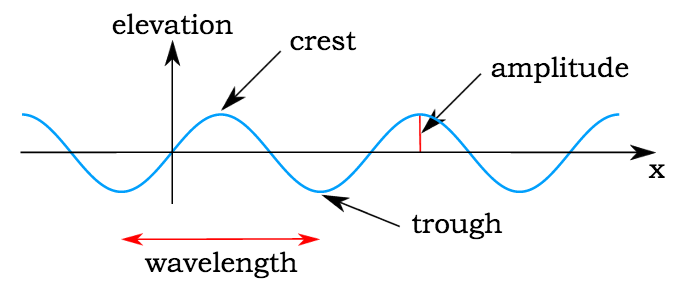Sinusoidal wave: This image shows the anatomy of a sine curve: the crest is the peak of each wave, and the trough is the valley; the amplitude is the distance between the crest and the x-axis; and the wavelength is the distance between two crests (or two troughs).

There are three measurable properties of wave motion: amplitude, wavelength, and frequency (the number of vibrations per second). The relation between the wavelength λ (Greek lambda) and frequency of a wave ν (Greek nu) is determined by the propagation velocity v, such that

$v=\nu \lambda$

For light, this equation becomes

$\nu = \frac{c}{\lambda}$

where c is the speed of light, 2.998 x 108 m/s.

When utilizing these equations to determine wavelength, frequency, or velocity by manipulation of the equation, it is important to note that wavelengths are expressed in units of length, such as meters, centimeters, nanometers, etc; and frequency is typically expressed as megahertz or hertz (s–1).

### Example

What is the wavelength of the musical note A = 440 hz when it is propagated through air in which the velocity of sound is 343 m s–1?

λ = v (343 m s-1)/ v(440 s–1) = 0.780 m

### Young’s Double-Slit Experiment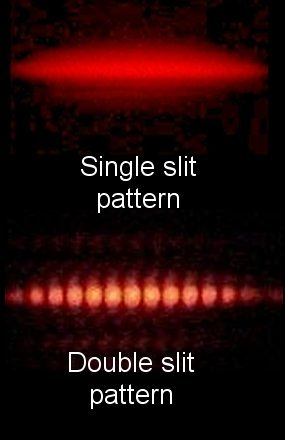Young’s double slit experiment: If light were purely a particle, it would not exhibit the interference pattern shown here.

In the early 19th century, English scientist Thomas Young carried out the famous double-slit experiment (also known as Young’s experiment), which demonstrated that a beam of light, when split into two beams and then recombined, will show interference effects that can only be explained by assuming that light is a wavelike disturbance. If light consisted strictly of ordinary or classical particles, and these particles were fired in a straight line through a slit and allowed to strike a screen on the other side, we would expect to see a pattern corresponding to the size and shape of the slit. However, when this single-slit experiment is actually performed, the pattern on the screen is a diffraction pattern in which the light is spread out. The smaller the slit, the greater the angle of spread.

Similarly, if light consisted strictly of classical particles and we illuminated two parallel slits, the expected pattern on the screen would simply be the sum of the two single-slit patterns. In actuality, however, the pattern changes to one with a series of alternating light and dark bands. When Thomas Young first demonstrated this phenomenon, it indicated that light consists of waves, as the distribution of brightness can be explained by the alternately additive and subtractive interference of wavefronts. Young’s experiment, performed in the early 1800’s, played a vital part in the acceptance of the wave theory of light, superseding the corpuscular theory of light proposed by Isaac Newton, which had been the accepted model of light propagation in the 17th and 18th centuries. Almost a century later, in 1905, Albert Einstein’s Nobel-Prize winning research into the photoelectric effect demonstrated that light can behave as if it is composed of discrete particles under certain conditions. These seemingly contradictory discoveries made it necessary to go beyond classical physics and take the quantum nature of light into account.

## Electromagnetic Spectrum

The electromagnetic spectrum is the range of all possible frequencies of electromagnetic radiation.

### Learning Objectives

Calculate frequency or photon energy, identify the three physical properties of electromagnetic waves

### Key Takeaways

#### Key Points

• The electromagnetic spectrum includes common regimes such as ultraviolet, visible, microwave, and radio waves.
• Electromagnetic waves are typically described by any of the following three physical properties: frequency (f), wavelength (λ), or intensity (I). Light quanta are typically described by frequency (f), wavelength (λ), or photon energy (E). The spectrum can be ordered according to frequency or wavelength.
• Electromagnetic radiation interacts with matter in different ways in different parts of the spectrum. The types of interaction can range from electronic excitation to molecular vibration depending on the different types of radiation, such as ultraviolet, X-rays, microwaves, and infrared radiation.

#### Key Terms

• gamma ray: Electromagnetic radiation of high frequency and therefore high energy per photon.
• spectrum: A range of colors representing light (electromagnetic radiation) of contiguous frequencies; hence electromagnetic spectrum, visible spectrum, ultraviolet spectrum, etc.
• photon: The quantum of light and other electromagnetic energy, regarded as a discrete particle having zero rest mass, no electric charge, and an indefinitely long lifetime.

### Range of the Electromagnetic Spectrum

The electromagnetic spectrum is the range of all possible frequencies of electromagnetic radiation. The electromagnetic spectrum of an object has a different meaning: it is the characteristic distribution of electromagnetic radiation emitted or absorbed by that particular object.Properties of the electromagnetic spectrum: The wavelengths of various regions of the electromagnetic spectrum are shown alongside an approximate proxy for size of the wavelength.

The electromagnetic spectrum extends from below the low frequencies used for modern radio communication to gamma radiation at the short-wavelength (high-frequency) end, covering wavelengths from thousands of kilometers down to a fraction of the size of an atom. The limit for long wavelengths is the size of the universe itself, while it is thought that the short wavelength limit is in the vicinity of the Planck length (1.616 x 10-35 m), although in principle the spectrum is infinite and continuous.

Most parts of the electromagnetic spectrum are used in science for spectroscopic and other probing interactions, as ways to study and characterize matter. In general, if the wavelength of electromagnetic radiation is of a similar size to that of a particular object (atom, electron, etc.), then it is possible to probe that object with that frequency of light. In addition, radiation from various parts of the spectrum has been found to have many other uses in communications and manufacturing.

### Energy of Photon

Electromagnetic waves are typically described by any of the following three physical properties: the frequency (f) (also sometimes represented by the Greek letter nu, ν), wavelength (λ), or photon energy (E). Frequencies observed in astronomy range from 2.4×1023 Hz (1 GeV gamma rays ) down to the local plasma frequency of the ionized interstellar medium (~1 kHz). Wavelength is inversely proportional to wave frequency; hence, gamma rays have very short wavelengths that are a fraction of the size of atoms, whereas other wavelengths can be as long as the universe. Photon energy is directly proportional to the wave frequency, so gamma ray photons have the highest energy (around a billion electron volts), while radio wave photons have very low energy (around a femto-electron volt). These relations are illustrated by the following equations:

$f = \frac{c}{\lambda} \,\,\,\text{ or }\,\,\, f= \frac{E}{h} \,\,\,\text{ or } \,\,\,E= \frac{hc}{\lambda}$

c = 299,792,458 m/s is the speed of light in vacuum

h = 6.62606896(33)×10−34 J s = 4.13566733(10)×10−15 eV s = Planck’s constant.

Whenever electromagnetic waves exist in a medium with matter, their wavelength is decreased. Wavelengths of electromagnetic radiation, no matter what medium they are traveling through, are usually quoted in terms of the vacuum wavelength, although this is not always explicitly stated. Generally, electromagnetic radiation is classified by wavelength into radio wave, microwave, terahertz (or sub-millimeter) radiation, infrared, the visible region we perceive as light, ultraviolet, X-rays, and gamma rays. The behavior of electromagnetic radiation depends on its wavelength. When electromagnetic radiation interacts with single atoms and molecules, its behavior also depends on the amount of energy per quantum (photon) it carries.

A.2.1 Describe the electromagnetic spectrum IB Chemistry SL – YouTube: This time with equations! Wave number = 1/wavelength in cm Speed of light = wavelength x frequency Energy = Planck’s constant x frequency. Dr Atkinson soon moved on to the un-needed gamma rays and improved them to delta rays!

### Interaction of Elecromagnetic Radiation with Matter

Electromagnetic radiation interacts with matter in different ways in different parts of the spectrum. The types of interaction can be so different that it seems justified to refer to different types of radiation. At the same time, there is a continuum containing all these different kinds of electromagnetic radiation. Thus, we refer to a spectrum, but divide it up based on the different interactions with matter. Below are the regions of the spectrum and their main interactions with matter:

• Radio: Collective oscillation of charge carriers in bulk material (plasma oscillation). An example would be the oscillation of the electrons in an antenna.
• Microwave through far infrared: Plasma oscillation, molecular rotation.
• Near infrared: Molecular vibration, plasma oscillation (in metals only).
• Visible: Molecular electron excitation (including pigment molecules found in the human retina), plasma oscillations (in metals only).
• Ultraviolet: Excitation of molecular and atomic valence electrons, including ejection of the electrons (photoelectric effect).
• X-rays: Excitation and ejection of core atomic electrons, Compton scattering (for low atomic numbers).
• Gamma rays: Energetic ejection of core electrons in heavy elements, Compton scattering (for all atomic numbers), excitation of atomic nuclei, including dissociation of nuclei.
• High-energy gamma rays: Creation of particle-antiparticle pairs. At very high energies, a single photon can create a shower of high-energy particles and antiparticles upon interaction with matter.

This classification goes in the increasing order of frequency and decreasing order of wavelength, which is characteristic of the type of radiation. While, in general, the classification scheme is accurate, in reality there is often some overlap between neighboring types of electromagnetic energy. For example, SLF radio waves at 60 Hz may be received and studied by astronomers, or may be ducted along wires as electric power, although the latter is, in the strict sense, not electromagnetic radiation at all.

## Interference and Diffraction

Interference and diffraction are terms that describe a wave interacting with something that changes its amplitude, such as another wave.

### Learning Objectives

Recognize the difference between constructive and destructive interference, and between interference and diffraction

### Key Takeaways

#### Key Points

• In physics, interference is a phenomenon in which two waves superimpose to form a resultant wave of greater or lower amplitude.
• Constructive interference occurs when the phase difference between the waves is a multiple of 2π, whereas destructive interference occurs when the difference is π, 3π, 5π, etc.
• Diffraction refers to various phenomena that occur when a wave encounters an obstacle. In classical physics, the diffraction phenomenon is described as the apparent bending of waves around small obstacles and the spreading out of waves past small openings.

#### Key Terms

• interference: An effect caused by the superposition of two systems of waves, such as a distortion on a broadcast signal due to atmospheric or other effects. In physics, interference is a phenomenon in which two waves superimpose to form a resultant wave of greater or lower amplitude.
• diffraction: The breaking up of an electromagnetic wave as it passes a geometric structure (e.g., a slit), followed by reconstruction of the wave by interference.
• amplitude: The maximum absolute value of some quantity that varies, especially a wave.

In physics, interference is a phenomenon in which two waves superimpose to form a resultant wave of greater or lower amplitude. Interference usually refers to the interaction of waves that are correlated or coherent with each other, either because they come from the same source or because they have the same (or nearly the same) frequency. Interference effects can be observed with all types of waves, including light, radio, acoustic, and surface water waves. In chemistry, the applications of interference to light are the most relevant to the study of matter.

### Mechanism of Interference

The principle of superposition of waves states that when two or more waves are incident on the same point, the total displacement at that point is equal to the vector sum of the displacements of the individual waves. If a crest of a wave meets a crest of another wave of the same frequency at the same point, then the magnitude of the displacement is the sum of the individual magnitudes; this is known as constructive interference. If a crest of one wave meets a trough of another wave, then the magnitude of the displacements is equal to the difference in the individual magnitudes; this is known as destructive interference.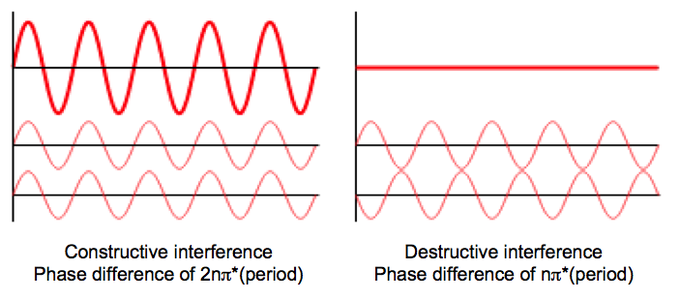Interference of two waves: These two examples represent constructive (left) and destructive interference (right) in wave phenomena. When the two waves are “in phase,” their periods are offset by 2nπ*period. However, when they are precisely out of phase, destructive interference results if the phase difference is nπ*period.

Constructive interference occurs when the phase difference between the waves is a multiple of 2π, whereas destructive interference occurs when the difference is π, 3π, 5π, etc. If the difference between the phases is intermediate between these two extremes, then the magnitude of the displacement of the summed waves lies between the minimum and maximum values.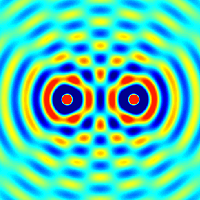Two Sources of Interference: The effect of two waves interfering with each other, for example, two stones thrown into a pool of water.

Consider, for example, what happens when two identical stones are dropped into a still pool of water at different locations. Each stone generates a circular wave propagating outwards from the point where the stone was dropped. When the two waves overlap, the net displacement at a particular point is the sum of the displacements of the individual waves. At some points, these will be in phase and will produce a maximum displacement. In other places, the waves will be in anti-phase and there will be no net displacement at these points. Thus, parts of the surface will be stationary.

### Diffraction

Diffraction refers to various phenomena that occur when a wave encounters an obstacle. In classical physics, the diffraction phenomenon is described as the apparent bending of waves around small obstacles and the spreading out of waves past small openings. Similar effects occur when light waves travel through a medium with a varying refractive index or a sound wave through one with varying acoustic impedance. Diffraction occurs with all waves, including sound waves, water waves, and electromagnetic waves such as visible light, X-rays, and radio waves. As physical objects have wave-like properties (at the atomic level), diffraction also occurs with matter and can be studied according to the principles of quantum mechanics. Italian scientist Francesco Maria Grimaldi coined the word diffraction and was the first to record accurate observations of the phenomenon in 1665.Diffraction: In classical physics, the diffraction phenomenon is described as the apparent bending of waves around small obstacles and the spreading out of waves past small openings.

The effects of diffraction are often seen in everyday life. The most striking examples of diffraction are those involving light; for example, the closely spaced tracks on a CD or DVD act as a diffraction grating to form the familiar rainbow pattern seen when looking at a disk. This principle can be extended to engineer a grating with a structure such that it will produce any diffraction pattern desired; the hologram on a credit card is an example. Diffraction in the atmosphere by small particles can cause a bright ring to be visible around a bright light source like the sun or the moon. A shadow of a solid object, using light from a compact source, shows small fringes near its edges. All these effects occur because light propagates as a wave.

Richard Feynman said, “No one has ever been able to define the difference between interference and diffraction satisfactorily. It is just a question of usage, and there is no specific, important physical difference between them.”

He suggested that when there are only a few sources, say two, we call it interference (as in Young’s slits), but with a large number of sources, the process can be labelled diffraction.

While diffraction occurs whenever propagating waves encounter such changes, its effects are generally most pronounced for waves where the wavelength is roughly similar to the dimensions of the diffracting objects. If the obstructing object provides multiple, closely spaced openings, a complex pattern of varying intensity can result. This is due to the superposition, or interference, of different parts of a wave that traveled to the observer by different paths (see diffraction grating).

## Planck’s Quantum Theory

Max Planck suggested that the energy of light is proportional to its frequency, also showing that light exists in discrete quanta of energy.

### Learning Objectives

Calculate the energy element E=hv, using Planck’s Quantum Theory

### Key Takeaways

#### Key Points

• Until the late 19th century, Newtonian physics dominated the scientific worldview. However, by the early 20th century, physicists discovered that the laws of classical mechanics do not apply at the atomic scale.
• The photoelectric effect could not be rationalized based on existing theories of light, as an increase in the intensity of light did not lead to the same outcome as an increase in the energy of the light.
• Planck postulated that the energy of light is proportional to the frequency, and the constant that relates them is known as Planck’s constant (h). His work led to Albert Einstein determining that light exists in discrete quanta of energy, or photons.

#### Key Terms

• photoelectric effect: The emission of electrons from the surface of a material following the absorption of electromagnetic radiation.
• electromagnetic radiation: Radiation (quantized as photons) consisting of oscillating electric and magnetic fields oriented perpendicularly to each other, moving through space.

In the late 18th century, great progress in physics had been made. Classical Newtonian physics at the time was widely accepted in the scientific community for its ability to accurately explain and predict many phenomena. However, by the early 20th century, physicists discovered that the laws of classical mechanics are not applicable at the atomic scale, and experiments such as the photoelectric effect completely contradicted the laws of classical physics. As a result of these observations, physicists articulated a set of theories now known as quantum mechanics. In some ways, quantum mechanics completely changed the way physicists viewed the universe, and it also marked the end of the idea of a clockwork universe (the idea that universe was predictable).

Electromagnetic (EM) radiation is a form of energy with both wave -like and particle-like properties; visible light being a well-known example. From the wave perspective, all forms of EM radiation may be described in terms of their wavelength and frequency. Wavelength is the distance from one wave peak to the next, which can be measured in meters. Frequency is the number of waves that pass by a given point each second. While the wavelength and frequency of EM radiation may vary, its speed in a vacuum remains constant at 3.0 x 108 m/sec, the speed of light. The wavelength or frequency of any specific occurrence of EM radiation determine its position on the electromagnetic spectrum and can be calculated from the following equation:

$c=\lambda\nu$

where c is the constant 3.0 x 108 m/sec (the speed of light in a vacuum), $\lambda$ = wavelength in meters, and $\nu$=frequency in hertz (1/s). It is important to note that by using this equation, one can determine the wavelength of light from a given frequency and vice versa.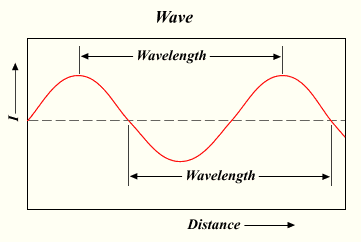Wavelength of EM radiation: The distance used to determine the wavelength is shown. Light has many properties associated with its wave nature, and the wavelength in part determines these properties.

### The Discovery of the Quantum

The wave model cannot account for something known as the photoelectric effect. This effect is observed when light focused on certain metals emits electrons. For each metal, there is a minimum threshold frequency of EM radiation at which the effect will occur. Replacement of light with twice the intensity and half the frequency will not produce the same outcome, contrary to what would be expected if light acted strictly as a wave. In that case, the effect of light would be cumulative—the light should add up, little by little, until it caused electrons to be emitted. Instead, there is a clear-cut minimum frequency of light that triggers electron ejection. The implication was that frequency is directly proportional to energy, with the higher light frequencies having more energy. This observation led to the discovery of the minimum amount of energy that could be gained or lost by an atom. Max Planck named this minimum amount the “quantum,” plural “quanta,” meaning “how much.” One photon of light carries exactly one quantum of energy.

Planck is considered the father of the Quantum Theory. According to Planck: E=h$\nu$, where h is Planck’s constant (6.62606957(29) x 10-34 J s), ν is the frequency, and E is energy of an electromagnetic wave. Planck (cautiously) insisted that this was simply an aspect of the processes of absorption and emission of radiation and had nothing to do with the physical reality of the radiation itself. However, in 1905, Albert Einstein reinterpreted Planck’s quantum hypothesis and used it to explain the photoelectric effect, in which shining light on certain materials can eject electrons from the material.

### More Evidence for a Particle Theory of Energy

When an electric current is passed through a gas, some of the electrons in the gas molecules move from their ground energy state to an excited state that is further away from their nuclei. When the electrons return to the ground state, they emit energy of various wavelengths. A prism can be used to separate the wavelengths, making them easy to identify. If light acted only as a wave, then there should be a continuous rainbow created by the prism. Instead, there are discrete lines created by different wavelengths. This is because electrons release specific wavelengths of light when moving from an excited state to the ground state.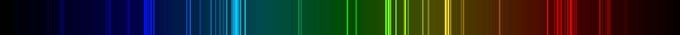Emission spectrum of nitrogen gas: Each wavelength of light emitted (each colored line) corresponds to a transition of an electron from one energy level to another, releasing a quantum of light with defined energy (color).

## The Photoelectric Effect

The photoelectric effect is the propensity of high-energy electromagnetic radiation to eject electrons from a given material.

### Learning Objectives

Explain the the photoelectric effect and understand its mathematical description

### Key Takeaways

#### Key Points

• In the photoelectric effect, electrons are emitted from matter (typically metals and non-metallic solids ) as a consequence of their absorption of energy from electromagnetic radiation of high frequency (short wavelength), such as ultraviolet light.
• When electromagnetic radiation interacts with an atom, it either excites electrons to a higher energy level known as an excited state, or, if the energy of the light is sufficiently high, it can ionize the atom by removing the electron.
• For a given metal, there exists a certain minimum frequency of incident radiation below which no photoelectrons are emitted. This frequency is called the threshold frequency.

#### Key Terms

• work function: The minimum energy needed to remove an electron from the surface of a material.
• stopping voltage: The voltage required to completely balance the kinetic energy of electrons ejected from a material’s surface.

In the photoelectric effect, electrons are emitted from matter (metals and non-metallic solids, liquids, or gases) as a consequence of their absorption of energy from electromagnetic radiation of high frequency (short wavelength), such as ultraviolet radiation. Electrons emitted in this manner may be referred to as photoelectrons. This phenomenon was first observed by Heinrich Hertz in 1887.The Photoelectric Effect: Electrons are emitted from matter by absorbed light.

The photoelectric effect has been demonstrated using light with energies from a few electronvolts (eV) to over 1 MeV in high atomic number elements. Study of the photoelectric effect led to an improved understanding of quantum mechanics as well as an appreciation of the wave-particle duality of light. It also led to Max Planck’s discovery of quanta (E=h$\nu$), which links frequency ($\nu$) with photon energy (E).

Planck’s constant, h, is also known as “the quantum of action.” It is a subatomic-scale constant and is one of the smallest constants used in physics. Other phenomena where light affects the movement of electric charges include the photoconductive effect (also known as photoconductivity or photoresistivity), the photovoltaic effect, and the photoelectrochemical effect.

### Emission Mechanism

All atoms have their electrons in orbitals with well-defined energy levels. When electromagnetic radiation interacts with an atom, it can excite the electron to a higher energy level, which can then fall back down, returning to the ground state. However, if the energy of the light is such that the electron is excited above energy levels associated with the atom, the electron can actually break free from the atom leading to ionization of the atom. This, in essence, is the photoelectric effect.

The photons of a beam of light have a characteristic energy proportional to the frequency of the light. In the photoemission process, if an electron within some material absorbs the energy of one photon and acquires more energy than the work function of the material (the electron binding energy), it is ejected. If the photon energy is too low, the electron is unable to escape the material. Increasing the intensity of the light increases the number of photons in the beam of light and thus increases the number of electrons excited but does not increase the energy that each electron possesses. The energy of the emitted electrons does not depend on the intensity of the incoming light (the number of photons), only on the energy or frequency of the individual photons. It is strictly an interaction between the incident photon and the outermost electron.

Electrons can absorb energy from photons when irradiated, but they usually follow an all-or-nothing principle. Typically, one photon is either energetic enough to cause emission of an electron or the energy is lost as the atom returns back to the ground state. If excess photon energy is absorbed, some of the energy liberates the electron from the atom and the rest contributes to the electron’s kinetic energy as a free particle.

### Experimental Observations of Photoelectric Emission

For a given metal, there exists a certain minimum frequency of incident radiation below which no photoelectrons are emitted. This frequency is called the threshold frequency. Increasing the frequency of the incident beam and keeping the number of incident photons fixed (resulting in a proportionate increase in energy) increases the maximum kinetic energy of the photoelectrons emitted. The number of electrons emitted also changes because the probability that each impacting photon results in an emitted electron is a function of the photon energy. However, if just the intensity of the incident radiation is increased, there is no effect on the kinetic energies of the photoelectrons.

For a given metal and frequency of incident radiation, the rate at which photoelectrons are ejected is directly proportional to the intensity of the incident light. An increase in the intensity of the incident beam (keeping the frequency fixed) increases the magnitude of the photoelectric current, though the stopping voltage remains the same. The time lag between the incidence of radiation and the emission of a photoelectron is very small, less than 10−9 second, and is unaffected by intensity changes.

### Mathematical Description

The maximum kinetic energy of an ejected electron is given by

$K.E._{max}=hf-\varphi$

where h is the Planck constant (6.626 x 10-34 m2kg/s) and f is the frequency of the incident photon. The term $\varphi$ is the work function (sometimes denoted W or ϕ), which gives the minimum energy required to remove a delocalized electron from the surface of the metal.

The work function satisfies $\varphi = hf_{0}$

where f0 is the threshold frequency for the metal. The maximum kinetic energy of an ejected electron is then

$K.E._{max} = h(f-f_0)$

Kinetic energy must be positive for ejection to take place, so we must have f > f0 for the photoelectric effect to occur.

### Photomultipliers

Photomultipliers are extremely light-sensitive vacuum tubes with a photocathode coated onto part (an end or side) of the inside of the envelope. The photocathode contains combinations of materials, such as caesium, rubidium, and antimony, specially selected to provide a low work function, so when illuminated by even very low levels of light, the photocathode readily releases electrons. By means of a series of electrodes (dynodes) at ever-higher potentials, these electrons are accelerated and substantially increased in number through secondary emission to provide a readily detectable output current. Photomultipliers are still commonly used wherever low levels of light must be detected.